## How to create drop down list with multiple selections or values in Excel?

By default, you can only select one item in a data validation drop-down list in Excel. How can you make multiple selections in the drop-down list as below gif shown? The methods in this article can help you solve the problem.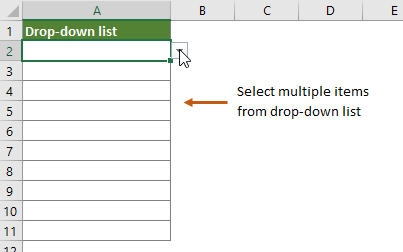#### Create drop down list with multiple selections with VBA code

This section provides two VBA codes to help you make multiple selections in drop-down lists in a worksheet. The two VBA codes can achieve:

VBA code 1: Allow multiple selections in a drop-down list without duplicates
VBA code 2: Allow multiple selections in a drop-down list without duplicates (delete existing items by selecting it again)

You can apply one of the following VBA codes to make multiple selections in a drop-down list in a worksheet in Excel. Please do as follows.

1. Open the worksheet contains the data validation drop-down lists from which you want to make multiple selections. Right click the sheet tab and select View Code from the context menu.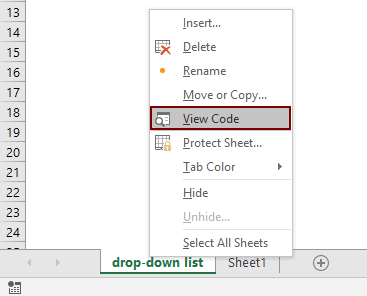2. In the Microsoft Visual Basic for Applications window, copy the below VBA code into the code window. See screenshot: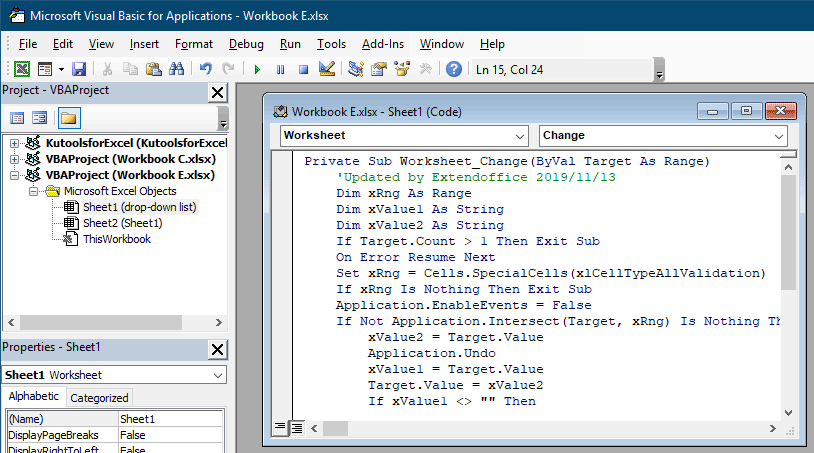VBA code 1: Allow multiple selections in a drop-down list without duplicates

``````Private Sub Worksheet_Change(ByVal Target As Range)
'Updated by Extendoffice 2019/11/13
Dim xRng As Range
Dim xValue1 As String
Dim xValue2 As String
If Target.Count > 1 Then Exit Sub
On Error Resume Next
Set xRng = Cells.SpecialCells(xlCellTypeAllValidation)
If xRng Is Nothing Then Exit Sub
Application.EnableEvents = False
If Not Application.Intersect(Target, xRng) Is Nothing Then
xValue2 = Target.Value
Application.Undo
xValue1 = Target.Value
Target.Value = xValue2
If xValue1 <> "" Then
If xValue2 <> "" Then
If xValue1 = xValue2 Or _
InStr(1, xValue1, ", " & xValue2) Or _
InStr(1, xValue1, xValue2 & ",") Then
Target.Value = xValue1
Else
Target.Value = xValue1 & ", " & xValue2
End If
End If
End If
End If
Application.EnableEvents = True
End Sub``````

3. Press the Alt + Q keys to close the Microsoft Visual Basic for Applications window.

Now you can select multiple items from the drop-down list in the current worksheet.Supposing you have selected an item accidently and need to remove it without clearing the whole cell and starting over. The following VBA code 2 can do you a favor.

VBA code 2: Allow multiple selections in a drop-down list without duplicates (delete existing items by selecting it again)

``````Private Sub Worksheet_Change(ByVal Target As Range)
'Updated by Extendoffice 2023/01/11
'Updated by Ken Gardner 2022/07/11
Dim xRng As Range
Dim xValue1 As String
Dim xValue2 As String
Dim semiColonCnt As Integer
Dim xType As Integer
If Target.Count > 1 Then Exit Sub
On Error Resume Next

xType = 0
xType = Target.Validation.Type
If xType = 3 Then
Application.ScreenUpdating = False
Application.EnableEvents = False
xValue2 = Target.Value
Application.Undo
xValue1 = Target.Value
Target.Value = xValue2
If xValue1 <> "" Then
If xValue2 <> "" Then
If xValue1 = xValue2 Or xValue1 = xValue2 & ";" Or xValue1 = xValue2 & "; " Then ' leave the value if only one in list
xValue1 = Replace(xValue1, "; ", "")
xValue1 = Replace(xValue1, ";", "")
Target.Value = xValue1
ElseIf InStr(1, xValue1, "; " & xValue2) Then
xValue1 = Replace(xValue1, xValue2, "") ' removes existing value from the list on repeat selection
Target.Value = xValue1
ElseIf InStr(1, xValue1, xValue2 & ";") Then
xValue1 = Replace(xValue1, xValue2, "")
Target.Value = xValue1
Else
Target.Value = xValue1 & "; " & xValue2
End If
Target.Value = Replace(Target.Value, ";;", ";")
Target.Value = Replace(Target.Value, "; ;", ";")
If Target.Value <> "" Then
If Right(Target.Value, 2) = "; " Then
Target.Value = Left(Target.Value, Len(Target.Value) - 2)
End If
End If
If InStr(1, Target.Value, "; ") = 1 Then ' check for ; as first character and remove it
Target.Value = Replace(Target.Value, "; ", "", 1, 1)
End If
If InStr(1, Target.Value, ";") = 1 Then
Target.Value = Replace(Target.Value, ";", "", 1, 1)
End If
semiColonCnt = 0
For i = 1 To Len(Target.Value)
If InStr(i, Target.Value, ";") Then
semiColonCnt = semiColonCnt + 1
End If
Next i
If semiColonCnt = 1 Then ' remove ; if last character
Target.Value = Replace(Target.Value, "; ", "")
Target.Value = Replace(Target.Value, ";", "")
End If
End If
End If
Application.EnableEvents = True
Application.ScreenUpdating = True
End If
End Sub
``````

Notes:

1). Duplicate values do not allow in the drop-down list.
2). The VBA code 2 above was provided by our enthusiastic user Ken Gardner on 2022/07/11.
3). Please save the workbook as an Excel Macro-Enabled Workbook in order to keep the code working in the future.
4). After adding the VBA code 2, you can remove an existing item by selecting it again in the drop-down list. See the gif below: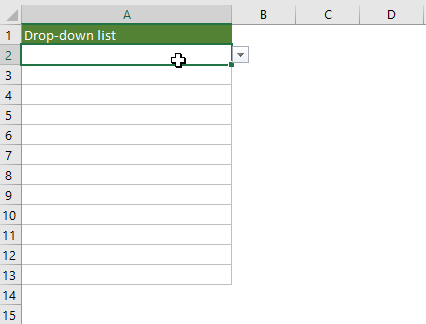#### Easily create drop down list with multiple selections with an amazing tool

As you can see, the above VBA codes are not easy to handle. Here we highly recommend the Multi-select Drop-down List feature of Kutools for Excel to help you easily handle this task with clicks. With this feature, you can easily select multiple items from the drop-down list in a specified range, current worksheet, current workbook or all opened workbooks as you need.

1. Select Kutools > Drop-down List > Multi-select Drop-down List.
2. In the Multi-select Drop-down List dialog box, do the following settings.
2.1) Specify where you want to apply this feature in the Set scope section;
2.2) Specify the separator for the selected items in a cell;
2.3) Decide the text direction；
2.4) Click OK.
3.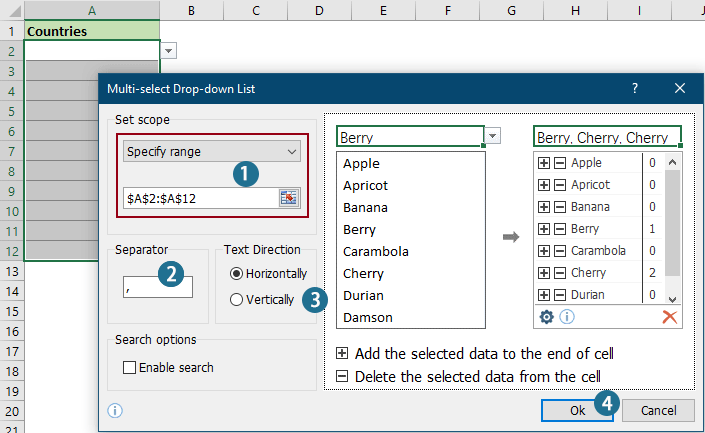##### Result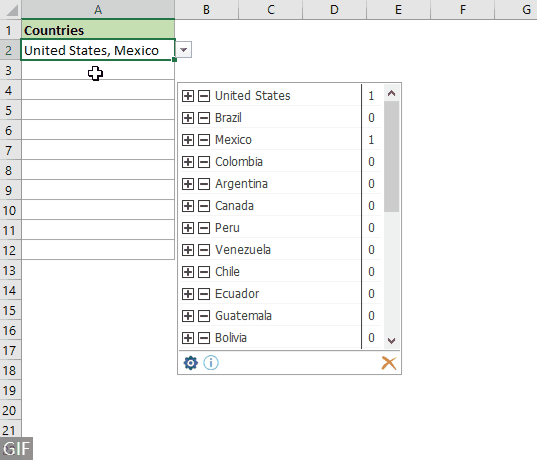#### Related articles:

Autocomplete when typing in Excel drop down list
If you have a data validation drop down list with large values, you need to scroll down in the list just for finding the proper one, or type the whole word into the list box directly. If there is method for allowing to auto complete when typing the first letter in the drop down list, everything will become easier. This tutorial provides the method to solve the problem.

Create drop down list from another workbook in Excel
It is quite easy to create a data validation drop down list among worksheets within a workbook. But if the list data you need for the data validation locates in another workbook, what would you do? In this tutorial, you will learn how to create a drop fown list from another workbook in Excel in details.

Create a searchable drop down list in Excel
For a drop down list with numerous values, finding a proper one is not an easy work. Previously we have introduced a method of auto completing drop down list when enter the first letter into the drop down box. Besides the autocomplete function, you can also make the drop down list searchable for enhancing the working efficiency in finding proper values in the drop down list. For making drop down list searchable, try the method in this tutorial.

Auto populate other cells when selecting values in Excel drop down list
Let’s say you have created a drop down list based on the values in cell range B8:B14. When you selecting any value in the drop down list, you want the corresponding values in cell range C8:C14 be automatically populated in a selected cell. For solving the problem, the methods in this tutorial will do you a favor.

More tutorial for drop down list...

### Best Office Productivity Tools

 Popular Features: Find, Highlight or Identify Duplicates   |  Delete Blank Rows   |  Combine Columns or Cells without Losing Data   |   Round without Formula ... Super Lookup: Multiple Criteria VLookup  |   Multiple Value VLookup  |   VLookup Across Multiple Sheets   |   Fuzzy Lookup .... Advanced Drop-down List: Quickly Create Drop Down List   |  Dependent Drop Down List   |  Multi-select Drop Down List .... Column Manager: Add a Specific Number of Columns  |  Move Columns  |  Toggle Visibility Status of Hidden Columns  |  Compare Ranges & Columns ... Featured Features: Grid Focus   |  Design View   |   Big Formula Bar   |  Workbook & Sheet Manager   |  Resource Library (Auto Text)   |  Date Picker   |  Combine Worksheets   |  Encrypt/Decrypt Cells   |  Send Emails by List   |  Super Filter   |   Special Filter (filter bold/italic/strikethrough...) ... Top 15 Toolsets:  12 Text Tools (Add Text, Remove Characters, ...)   |   50+ Chart Types (Gantt Chart, ...)   |   40+ Practical Formulas (Calculate age based on birthday, ...)   |   19 Insertion Tools (Insert QR Code, Insert Picture from Path, ...)   |   12 Conversion Tools (Numbers to Words, Currency Conversion, ...)   |   7 Merge & Split Tools (Advanced Combine Rows, Split Cells, ...)   |   ... and more

Supercharge Your Excel Skills with Kutools for Excel, and Experience Efficiency Like Never Before. Kutools for Excel Offers Over 300 Advanced Features to Boost Productivity and Save Time.  Click Here to Get The Feature You Need The Most...#### Office Tab Brings Tabbed interface to Office, and Make Your Work Much Easier

• Enable tabbed editing and reading in Word, Excel, PowerPoint, Publisher, Access, Visio and Project.
• Open and create multiple documents in new tabs of the same window, rather than in new windows.
• Increases your productivity by 50%, and reduces hundreds of mouse clicks for you every day!No ratings yet. Be the first to rate!
This comment was minimized by the moderator on the site
Hi, please guide me how I can merge the following two VBA Sheet codes (no in Module).
Thanks

Code 01:
``````Private Sub Worksheet_Change(ByVal Target As Range)
'Updated by Extendoffice 2019/11/13
Dim xRng As Range
Dim xValue1 As String
Dim xValue2 As String
If Target.Count > 1 Then Exit Sub
On Error Resume Next
Set xRng = Cells.SpecialCells(xlCellTypeAllValidation)
If xRng Is Nothing Then Exit Sub
Application.EnableEvents = False
If Not Application.Intersect(Target, xRng) Is Nothing Then
xValue2 = Target.Value
Application.Undo
xValue1 = Target.Value
Target.Value = xValue2
If xValue1 <> "" Then
If xValue2 <> "" Then
If xValue1 = xValue2 Or _
InStr(1, xValue1, ", " & xValue2) Or _
InStr(1, xValue1, xValue2 & ",") Then
Target.Value = xValue1
Else
Target.Value = xValue1 & ", " & xValue2
End If
End If
End If
End If
Application.EnableEvents = True
End Sub``````

Code 02:

``````Private Sub Worksheet_Change(ByVal Target As Range)
If Not Intersect(Target, Range("D1")) Is Nothing Then Filter_namebakhsh Range("D1").Value

If Not Intersect(Target, Range("F1")) Is Nothing Then Filter_saleshoroo Range("F1").Value

If Not Intersect(Target, Range("H1")) Is Nothing Then Filter_salekhatameh Range("H1").Value

End Sub``````
This comment was minimized by the moderator on the site
Bonjour,

Dans une cellule où apparaitrait plusieurs choix de réponses, comment peut-on faire pour qu'il y ait un retour à la ligne pour chacun des choix?
This comment was minimized by the moderator on the site
Hi LeRomain,
Try the following code. Hope it can help.

``````Private Sub Worksheet_Change(ByVal Target As Range)
'Updated by Extendoffice 2022/12/23
'Updated by Ken Gardner 2022/07/11
Dim xRng As Range
Dim xValue1 As String
Dim xValue2 As String
Dim semiColonCnt As Integer
If Target.Count > 1 Then Exit Sub
On Error Resume Next
Set xRng = Cells.SpecialCells(xlCellTypeAllValidation)
If xRng Is Nothing Then Exit Sub
Application.EnableEvents = False
'If Not Application.Intersect(Target, xRng) Is Nothing Then
If Application.Intersect(Target, xRng) Then
xValue2 = Target.Value
Application.Undo
xValue1 = Target.Value
Target.Value = xValue2
If xValue1 <> "" Then
If xValue1 = xValue2 Then
Target.Value = ""
ElseIf xValue2 <> "" Then
If xValue1 = xValue2 Or xValue1 = xValue2 & ";" Or xValue1 = xValue2 & "; " Then ' leave the value if only one in list
xValue1 = Replace(xValue1, vbLf, "")
xValue1 = Replace(xValue1, vbLf, "")
Target.Value = xValue1
ElseIf InStr(1, xValue1, vbLf & xValue2) Then
xValue1 = Replace(xValue1, vbLf & xValue2, "")  ' removes existing value from the list on repeat selection
Target.Value = xValue1
ElseIf InStr(1, xValue1, xValue2 & vbLf) Then
xValue1 = Replace(xValue1, xValue2, "")
Target.Value = xValue1
Else
Target.Value = xValue1 & vbLf & xValue2
End If
Target.Value = Replace(Target.Value, ";;", vbLf)
Target.Value = Replace(Target.Value, "; ;", vbLf)
If InStr(1, Target.Value, vbLf) = 1 Then  ' check for ; as first character and remove it
Target.Value = Replace(Target.Value, vbLf, "", 1, 1)
End If
If InStr(1, Target.Value, vbLf) = 1 Then
Target.Value = Replace(Target.Value, vbLf, "", 1, 1)
End If
semiColonCnt = 0
For i = 1 To Len(Target.Value)
If InStr(i, Target.Value, vbLf) Then
semiColonCnt = semiColonCnt + 1
End If
Next i
If semiColonCnt = 1 Then ' remove ; if last character
Target.Value = Replace(Target.Value, vbLf, "")
Target.Value = Replace(Target.Value, vbLf, "")
End If
End If
End If
End If
Application.EnableEvents = True
End Sub``````
This comment was minimized by the moderator on the site
Bonjour,
Si dans une cellule je souhaite que pour chacun des différents choix sélectionnés il y ait un retour à la ligne, comment faut-il faire?
This comment was minimized by the moderator on the site
(à l'attention de cristal)
Bonjour,

La macro fonctionne mais il me reste un dernier souci : Je voudrais que la macro fonctionne uniquement dans les colonnes V,W,X. J'ai vu que le sujet avait déjà été traité mais j'ignore quelles modifications apporter dans la mise à jour que vous venez de faire. Pouvez-vous apporter les modifications nécessaires s'il vous plaît ?

Merci.
This comment was minimized by the moderator on the site
Hi Said,

You just need to add the following line:
If Not (Target.Column > 21 And Target.Column < 25) Then Exit Sub
between the line "On Error Resume Next" and the line "xType = 0" line.
The entire VBA script is as follows:
``````Private Sub Worksheet_Change(ByVal Target As Range)
'Updated by Extendoffice 2023/01/12
'Updated by Ken Gardner 2022/07/11
Dim xRng As Range
Dim xValue1 As String
Dim xValue2 As String
Dim semiColonCnt As Integer
Dim xType As Integer
If Target.Count > 1 Then Exit Sub
On Error Resume Next

If Not (Target.Column > 21 And Target.Column < 25) Then Exit Sub

xType = 0
xType = Target.Validation.Type
If xType = 3 Then
Application.ScreenUpdating = False
Application.EnableEvents = False
xValue2 = Target.Value
Application.Undo
xValue1 = Target.Value
Target.Value = xValue2
If xValue1 <> "" Then
If xValue2 <> "" Then
If xValue1 = xValue2 Or xValue1 = xValue2 & ";" Or xValue1 = xValue2 & "; " Then ' leave the value if only one in list
xValue1 = Replace(xValue1, "; ", "")
xValue1 = Replace(xValue1, ";", "")
Target.Value = xValue1
ElseIf InStr(1, xValue1, "; " & xValue2) Then
xValue1 = Replace(xValue1, xValue2, "") ' removes existing value from the list on repeat selection
Target.Value = xValue1
ElseIf InStr(1, xValue1, xValue2 & ";") Then
xValue1 = Replace(xValue1, xValue2, "")
Target.Value = xValue1
Else
Target.Value = xValue1 & "; " & xValue2
End If
Target.Value = Replace(Target.Value, ";;", ";")
Target.Value = Replace(Target.Value, "; ;", ";")
If Target.Value <> "" Then
If Right(Target.Value, 2) = "; " Then
Target.Value = Left(Target.Value, Len(Target.Value) - 2)
End If
End If
If InStr(1, Target.Value, "; ") = 1 Then ' check for ; as first character and remove it
Target.Value = Replace(Target.Value, "; ", "", 1, 1)
End If
If InStr(1, Target.Value, ";") = 1 Then
Target.Value = Replace(Target.Value, ";", "", 1, 1)
End If
semiColonCnt = 0
For i = 1 To Len(Target.Value)
If InStr(i, Target.Value, ";") Then
semiColonCnt = semiColonCnt + 1
End If
Next i
If semiColonCnt = 1 Then ' remove ; if last character
Target.Value = Replace(Target.Value, "; ", "")
Target.Value = Replace(Target.Value, ";", "")
End If
End If
End If
Application.EnableEvents = True
Application.ScreenUpdating = True
End If
End Sub
``````
This comment was minimized by the moderator on the site
Bonjour Cristal,

Peux-tu me dire quelles lignes de code il faut ajouter pour que la macro fonctionne dans plusieurs ensemble de colonnes stp ?
(exemple : la macro fonctionne dans les colonnes A,B,C et F,G,H et O,P,Q etc.

Merci
This comment was minimized by the moderator on the site
Bonjour Cristal,

Je suis vraiment désolé de te demander autant mais j'aurai une dernière requête …
J'aimerai que dans la colonne D par exemple, les choix s'affichent sur une nouvelle ligne sans changer la configuration des colonnes V,W,X.
J'ai vu qu'il fallait ajouter vBNewLine pour cela mais encore une fois je ne sais où l'insérer dans le code.
Pourrais-tu m'aider s'il te plaît ?

Merci
This comment was minimized by the moderator on the site
(A l'attention de Cristal)
Bonjour,

Je poste un nouveau commentaire car quand je réponds à un commentaire ça ne le publie pas.
La macro fonctionne bien mais il me reste un dernier souci : Je voudrais que la macro ne fonctionne que dans les colonnes V,W et X. J'ai vu que ce sujet avait été traité mais les modifications n'ont pas l'air de fonctionner quand j'essaie. Pouvez-vous m'apporter les modifications nécessaires s'il vous plaît ?

Merci
This comment was minimized by the moderator on the site
Bonjour,

J'ai un petit problème.
La macro fonctionne bien mais le problème est que les formules de base ne fonctionnent plus sur la feuille. Quand je fais une formule ça me donne bien le résultat mais le contenu de la cellule se transforme en résultat aussi (par exemple le résultat de ma formule est 1, quand je clique sur la cellule le contenu est 1 et non la formule).
Pouvez-vous m'apporter la modification pour ce problème svp ? (J'ai essayé de faire la modif pour que la macro fonctionne que sur certaines colonnes mais ça a pas l'air de fonctionner...)

PS : J'avais aussi le problème du point virgule qui restait quand on désélectionnait un choix, problème qui a été résolu plus haut dans les commentaires, pouvez-vous prendre en compte ce point aussi dans votre réponse svp ?

Merci.
This comment was minimized by the moderator on the site
Hi Said,

Sorry for the inconvenience. The code has been modified and updated in the post. Please give it a try. Thank you for your feedback.
``````Private Sub Worksheet_Change(ByVal Target As Range)
'Updated by Extendoffice 2023/01/11
'Updated by Ken Gardner 2022/07/11
Dim xRng As Range
Dim xValue1 As String
Dim xValue2 As String
Dim semiColonCnt As Integer
Dim xType As Integer
If Target.Count > 1 Then Exit Sub
On Error Resume Next

xType = 0
xType = Target.Validation.Type
If xType = 3 Then
Application.ScreenUpdating = False
Application.EnableEvents = False
xValue2 = Target.Value
Application.Undo
xValue1 = Target.Value
Target.Value = xValue2
If xValue1 <> "" Then
If xValue2 <> "" Then
If xValue1 = xValue2 Or xValue1 = xValue2 & ";" Or xValue1 = xValue2 & "; " Then ' leave the value if only one in list
xValue1 = Replace(xValue1, "; ", "")
xValue1 = Replace(xValue1, ";", "")
Target.Value = xValue1
ElseIf InStr(1, xValue1, "; " & xValue2) Then
xValue1 = Replace(xValue1, xValue2, "") ' removes existing value from the list on repeat selection
Target.Value = xValue1
ElseIf InStr(1, xValue1, xValue2 & ";") Then
xValue1 = Replace(xValue1, xValue2, "")
Target.Value = xValue1
Else
Target.Value = xValue1 & "; " & xValue2
End If
Target.Value = Replace(Target.Value, ";;", ";")
Target.Value = Replace(Target.Value, "; ;", ";")
If Target.Value <> "" Then
If Right(Target.Value, 2) = "; " Then
Target.Value = Left(Target.Value, Len(Target.Value) - 2)
End If
End If
If InStr(1, Target.Value, "; ") = 1 Then ' check for ; as first character and remove it
Target.Value = Replace(Target.Value, "; ", "", 1, 1)
End If
If InStr(1, Target.Value, ";") = 1 Then
Target.Value = Replace(Target.Value, ";", "", 1, 1)
End If
semiColonCnt = 0
For i = 1 To Len(Target.Value)
If InStr(i, Target.Value, ";") Then
semiColonCnt = semiColonCnt + 1
End If
Next i
If semiColonCnt = 1 Then ' remove ; if last character
Target.Value = Replace(Target.Value, "; ", "")
Target.Value = Replace(Target.Value, ";", "")
End If
End If
End If
Application.EnableEvents = True
Application.ScreenUpdating = True
End If
End Sub``````
This comment was minimized by the moderator on the site
Bonjour,

La macro fonctionne mais il me reste un dernier souci : Je voudrais que la macro fonctionne uniquement dans les colonnes V,W,X. J'ai vu que le sujet avait déjà été traité mais j'ignore quelles modifications apporter dans la mise à jour que vous venez de faire. Pouvez-vous apporter les modifications nécessaires s'il vous plaît ?

Merci.
This comment was minimized by the moderator on the site
Bonjour,

Tout fonctionne bien merci !
Cependant il me reste un dernier problème : Je voudrais que le macro ne fonctionne que dans les colonnes V,W,X. J'ai vu que cette question avait été posée auparavant mais les modifications que j'apporte n'ont pas l'air de fonctionner. Pouvez-vous apporter les modifications nécessaires s'il vous plaît ?

Merci
This comment was minimized by the moderator on the site
Bonjour,
Tout fonctionne parfaitement merci !
Mais il me reste un dernier petit souci : je voudrais que la macro ne fonctionne que dans les colonnes V,W,X. Pouvez-vous apporter la modification nécessaire s'il vous plaît ?
J'ai vu que cette question avait déjà été posée mais ça ne fonctionne pas quand j'apporte les modifications qui ont été données.

Merci.
This comment was minimized by the moderator on the site
Hallo, ich hoffe es kann mir geholfen werden:
Ich habe mir den VBA-Code 2 in meiner Tabelle hinterlegt um eine Mehrfachauswahl in einigen Zellen zu treffen.
Wenn ich allerdings mein Blatt schütze funktioniert die Mehrfachauswahl nicht mehr und es wird immer nur der jeweilige Wert eingefügt, den ich gerade anklicke und der vorherige gelöscht/überschrieben. Ich habe mich jetzt schon mehrere Tage durch´s Web gegoogelt, aber nicht das richtige als Abhilfe gefunden. Hat evtl. jemand einen Rat bzw. Tipp für mich???
Grüße, Marko
This comment was minimized by the moderator on the site
Hi,

The following VBA code can help you solve the problem. Before protecting the worksheet, you need to unlock the cells containing the data validation drop-down list.
If you are not good at handling VBA code, the third-party tool recommended in the post can help in a protected worksheet.
``````Private Sub Worksheet_Change(ByVal Target As Range)
'Updated by Extendoffice 2022/12/23
'Updated by Ken Gardner 2022/07/11
Dim xRng As Range
Dim xValue1 As String
Dim xValue2 As String
Dim semiColonCnt As Integer
Dim xType As Integer
If Target.Count > 1 Then Exit Sub

On Error Resume Next

'    Set xRng = Cells.SpecialCells(xlCellTypeAllValidation)
'    If xRng Is Nothing Then Exit Sub

'        If Application.Intersect(Target, xRng) Then
xType = 0
xType = Target.Validation.Type
If xType = 3 Then
Application.EnableEvents = False
xValue2 = Target.Value
Application.Undo
xValue1 = Target.Value
Target.Value = xValue2
If xValue1 <> "" Then
If xValue2 <> "" Then
If xValue1 = xValue2 Or xValue1 = xValue2 & ";" Or xValue1 = xValue2 & "; " Then ' leave the value if only one in list
xValue1 = Replace(xValue1, "; ", "")
xValue1 = Replace(xValue1, ";", "")
Target.Value = xValue1
ElseIf InStr(1, xValue1, "; " & xValue2) Then
xValue1 = Replace(xValue1, xValue2, "") ' removes existing value from the list on repeat selection
Target.Value = xValue1
ElseIf InStr(1, xValue1, xValue2 & ";") Then
xValue1 = Replace(xValue1, xValue2, "")
Target.Value = xValue1
Else
Target.Value = xValue1 & "; " & xValue2
End If
Target.Value = Replace(Target.Value, ";;", ";")
Target.Value = Replace(Target.Value, "; ;", ";")
If InStr(1, Target.Value, "; ") = 1 Then ' check for ; as first character and remove it
Target.Value = Replace(Target.Value, "; ", "", 1, 1)
End If
If InStr(1, Target.Value, ";") = 1 Then
Target.Value = Replace(Target.Value, ";", "", 1, 1)
End If
semiColonCnt = 0
For i = 1 To Len(Target.Value)
If InStr(i, Target.Value, ";") Then
semiColonCnt = semiColonCnt + 1
End If
Next i
If semiColonCnt = 1 Then ' remove ; if last character
Target.Value = Replace(Target.Value, "; ", "")
Target.Value = Replace(Target.Value, ";", "")
End If
End If
End If
Application.EnableEvents = True
End If

End Sub``````
This comment was minimized by the moderator on the site
Bonjour,
Dans le Code VBA 2 : Autoriser plusieurs sélections dans une liste déroulante sans doublons (supprimer les éléments existants en les sélectionnant à nouveau), je souhaiterai que les sélections s'affiche avec saut de ligne et non pas à la suite, séparé par un point virgule ";".
Savez vous que faut il changer dans le code ?
Merci par avance,
Cordialement,
This comment was minimized by the moderator on the site
Hi PaulM,

The following VBA code can do you a favor, please give it a try. Thank you.
``````Private Sub Worksheet_Change(ByVal Target As Range)
'Updated by Extendoffice 2022/12/23
'Updated by Ken Gardner 2022/07/11
Dim xRng As Range
Dim xValue1 As String
Dim xValue2 As String
Dim semiColonCnt As Integer
If Target.Count > 1 Then Exit Sub
On Error Resume Next
Set xRng = Cells.SpecialCells(xlCellTypeAllValidation)
If xRng Is Nothing Then Exit Sub
Application.EnableEvents = False
'If Not Application.Intersect(Target, xRng) Is Nothing Then
If Application.Intersect(Target, xRng) Then
xValue2 = Target.Value
Application.Undo
xValue1 = Target.Value
Target.Value = xValue2
If xValue1 <> "" Then
If xValue1 = xValue2 Then
Target.Value = ""
ElseIf xValue2 <> "" Then
If xValue1 = xValue2 Or xValue1 = xValue2 & ";" Or xValue1 = xValue2 & "; " Then ' leave the value if only one in list
xValue1 = Replace(xValue1, vbLf, "")
xValue1 = Replace(xValue1, vbLf, "")
Target.Value = xValue1
ElseIf InStr(1, xValue1, vbLf & xValue2) Then
xValue1 = Replace(xValue1, vbLf & xValue2, "")  ' removes existing value from the list on repeat selection
Target.Value = xValue1
ElseIf InStr(1, xValue1, xValue2 & vbLf) Then
xValue1 = Replace(xValue1, xValue2, "")
Target.Value = xValue1
Else
Target.Value = xValue1 & vbLf & xValue2
End If
Target.Value = Replace(Target.Value, ";;", vbLf)
Target.Value = Replace(Target.Value, "; ;", vbLf)
If InStr(1, Target.Value, vbLf) = 1 Then  ' check for ; as first character and remove it
Target.Value = Replace(Target.Value, vbLf, "", 1, 1)
End If
If InStr(1, Target.Value, vbLf) = 1 Then
Target.Value = Replace(Target.Value, vbLf, "", 1, 1)
End If
semiColonCnt = 0
For i = 1 To Len(Target.Value)
If InStr(i, Target.Value, vbLf) Then
semiColonCnt = semiColonCnt + 1
End If
Next i
If semiColonCnt = 1 Then ' remove ; if last character
Target.Value = Replace(Target.Value, vbLf, "")
Target.Value = Replace(Target.Value, vbLf, "")
End If
End If
End If
End If
Application.EnableEvents = True
End Sub``````
This comment was minimized by the moderator on the site
Hola! Una pregunta: es posible limitar este comportamiento solo al rango de celdas en el que tengo las listas desplegables? Cuando introduzco un dato en cualquier celda que ya contiene un dato, en lugar de sobrescribirme el dato lo mantiene y añade el nuevo. No quiero que esto ocurra en todas las celdas sino solo en las listas desplegables. Gracias!
This comment was minimized by the moderator on the site
Hi Carlos,
I don't quite understand what you mean. The methods provided in the post are only work with cells that have drop-down lists.
This comment was minimized by the moderator on the site
Hello!

Two Questions;

How do I get this code to just apply to Column 'A' of my workbook?

If I want to filter the multiple selections I have in the box, for example if I have "blue; red; yellow; green" in one box and the one below has "yellow; green" and I only want to select the boxes with green in it how do I go about doing that? Right now if I filter the content and I search for just green, the "yellow; green box" will not appear because it is paired with another value but I want to be able to search for it regardless of the values it is paired with.
This comment was minimized by the moderator on the site
Hi Chase,
1) If you want to just apply to Column A of your workbook using the VBA method. You can try the following VBA code.
2) For second question, use the Filter function in Excel can help to filter all the boxes with green in it.

``````Private Sub Worksheet_Change(ByVal Target As Range)
'Updated by Extendoffice 20220929
Dim xRng As Range
Dim xValue1 As String
Dim xValue2 As String
If Target.Count > 1 Then Exit Sub
On Error Resume Next
Set xRng = Application.Range("A1:A500")
If xRng Is Nothing Then Exit Sub
Application.EnableEvents = False
If Not Application.Intersect(Target, xRng) Is Nothing Then
xValue2 = Target.Value
Application.Undo
xValue1 = Target.Value
Target.Value = xValue2
If xValue1 <> "" Then
If xValue2 <> "" Then
If xValue1 = xValue2 Or _
InStr(1, xValue1, ", " & xValue2) Or _
InStr(1, xValue1, xValue2 & ",") Then
Target.Value = xValue1
Else
Target.Value = xValue1 & ", " & xValue2
End If
End If
End If
End If
Application.EnableEvents = True
End Sub``````
There are no comments posted here yet